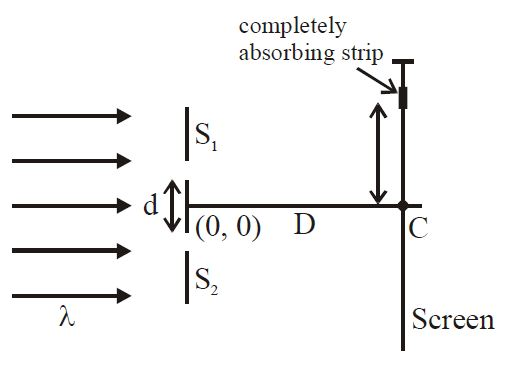# Force on Strip in YDSE!Figure shows two identical narrow slits $S_1$ and $S_2$.

A very small completely absorbing strip is placed at distance '$y$' from the point $C$.

'$C$' is the point on the screen equidistant from $S_1$ and $S_2$.

Assume ($\lambda << d << D$) where $\lambda, d$ and $D$ have usual meaning.

When $S_2$ is covered, the force due to light acting on strip is '$F$' and when both slits are opened the force acting on strip is $2F$. Minimum positive '$y$' ($<) coordinate of the strip in terms of $\lambda, d$ and $D$ is $\frac{\lambda D}{2md}$.

What is the value of $m$?

×